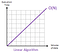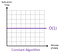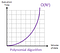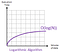# Big Ohhhhh Notation 🤦🏽Source: Pixabay

If you’re haven’t come across the Big O during your programming journey, you surely will at some point. When I first stumbled upon it, I was completely dumbfounded! However, reading more into it and working with examples, it was a major face palm moment. In this blog, I’ll be discussing Big O Notation and looking at a few basic aspects that can help you dive deeper into more advanced areas of it.

## Why Big and Why O? 🤔

Computer scientists borrowed this term from the world of mathematics to describe a concise language around the efficiency of algorithms and data structures. Do not worry though, I’ll leave out the mathematical patois when describing this concept and the concepts within.

Big O Notation refers to the time complexity of an algorithm. It allows us to identify the efficiency of algorithms and how we can improve the way we approach them.

## Step by Step 🪜

Big O aims to focus on the number of steps in relation to the number of elements that are given. So, logic would conclude that if the number of steps taken is far less than the number of elements that are dealt with in that algorithm, this would be an efficient algorithm. However, the opposite scenario also creates the opposite result and there would also be a middle point, where the number of steps is the same as the number of elements. Finally, you can/will encounter a scenario where, no matter the number of elements, the algorithm will always take the same number of steps.

## Big O of N — O of N — O(n) 📏

Remember, that the best way to describe the Big O is the number of steps taken to complete an algorithm in relation to the number of elements provided. Or in other words, if there are N number of elements, how many steps will the algorithm take?

Firstly, we will be looking at O(n) (pronounced as either heading above). O(n) tells us that an algorithm will be completed in the same amount of steps as there are elements. So, if there are 10 elements, it would take 10 steps to complete the algorithm. If there are 20 elements, it would take 20 steps. If there are 30 elements, it would take 30 steps. You get the picture.

Speaking of pictures, let’s take a look at this via a graph:Source: https://www.101computing.net/big-o-notation/

The y axis (execution time) refers to the number of steps and the x axis (volume of data) refers to the number of elements. As you can see, the number of steps grows in direct proportion to the size of the elements being handled and therefore known as a linear algorithm.

Here’s an example of a JavaScript function that shows O(n):

`function arrSum(array) {  let sum = 0;    for (let i = 0; i < array; i++) {    sum += array[i];  }    return sum;}`

## Big O of 1— O of 1— O(1) ☝🏽

Now we will be looking at O(1) (pronounced as either heading above). O(1) tells us that an algorithm will be completed in the same number of steps no matter the number of elements. So, if there are 10 elements, it would take 5 steps to complete the algorithm. If there are 20 elements, it would also take 5 steps. If there are 30 elements, it would again take 5 steps. So, the ‘1’ is referring to the consistent number of steps, not necessarily ‘1’ step.

Let’s visualise it on a graph:Source: https://www.101computing.net/big-o-notation/

As you can see, this graph demonstrates that an algorithm will always execute in the same amount of execution time/number of steps regardless of the size of the elements/data set.

Here’s an example of a JavaScript function that shows O(1):

`function isLeapYear(year) {  return (year % 100 === 0) ? (year % 400 === 0) : (year % 4 === 0);}`

No matter what year is inputted, 1990 or 2021, the algorithm will not vary in how many steps it takes and thus being O(1).

## Big O of N Squared— O of N Squared— O(n²) 🔲

Here we will be looking at O(n²) (pronounced as either heading above). O(n²) tells us that an algorithm will be completed in the squared number of steps in relation to the elements. So, if there are 10 elements, it would take 100 steps to complete the algorithm. If there are 20 elements, it would take 400 steps. If there are 30 elements, it would take 900 steps. This notation shows us exponential growth of steps and is extremely inefficient.

Let’s see how it would be represented on a graph:Source: https://www.101computing.net/big-o-notation/

O(n²) represents performance that is directly proportional to the square of the size of the elements. As the elements increase by 1, there would be rapid growth of steps.

Usually, not all the time, nested loops are O(n²). Here’s an example of a JavaScript nested loop that could be O(n²):

`for (int i = 0; i < n; i++) {    for (int j = 0; j < n; j++) {      // Your code    }}`

## Big O of Log N — O of Log N— O(log n) 🪵

Finally, we will be looking at O(log n) (pronounced as either heading above). You may have heard of logarithms and O(log n) refers to just that. A logarithm is represented as log2 n but in computer science we omit the 2, making it just O(log n). So, O(log n) means that for N number of element in an algorithm, it would take log2 n steps. If there were 8 elements to deal with in an algorithm, it would take three steps to execute as log2 8 = 3 or in other words you would have to divide 8 by 2, 3 times, to get 1 (8 / 2 / 2 / 2 = 1). This is an efficient algorithm as the number of steps is far less than the number of elements.

Let’s see this on a graph:Source: https://www.101computing.net/big-o-notation/

A logarithmic algorithm O(log(N)) expresses growth decreasing when the elements increase following a logarithmic curve. Logarithmic algorithms are hence quite efficient especially when processing large sets of data.

Here’s an example of a JavaScript function that shows O(log n):

`function chessBoardSpace(numberOfGrains) {  let chessBoardSpaces = 1;  let placedGrains = 1;  while (placedGrains < numberOfGrains) {    placedGrains *=2;    chessBoardSpaces +=1;  }  return chessBoardsSpaces;}`

This function calculates which square, on a chess board, you will need to place a particular number of grains. For example, when you input 16 as the `numberOfGrains`, this would return 5 as you would place 16 grains on the 5th square. As you can see, for each next square, the number of grains doubles and so the number of steps taken to complete the algorithm is far less than the elements.

I hope you were able to learn about the different aspects of Big O Notation and how our algorithms play a huge role in efficiency. May you journey with the Big O be a steady one!

## Star Gazers

“If you want to master something, teach it.”

## Star Gazers

The place that enthusiastic and stargazer to everything.

Written by

## Milan Parmar

Software Engineer 👨🏽‍💻 | Full Stack Web Development 💻 | Smartphone Tech Enthusiast📱## Star Gazers

The place that enthusiastic and stargazer to everything.

## More From Medium

Medium is an open platform where 170 million readers come to find insightful and dynamic thinking. Here, expert and undiscovered voices alike dive into the heart of any topic and bring new ideas to the surface. Learn more

Follow the writers, publications, and topics that matter to you, and you’ll see them on your homepage and in your inbox. Explore

If you have a story to tell, knowledge to share, or a perspective to offer — welcome home. It’s easy and free to post your thinking on any topic. Write on Medium